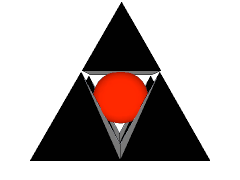Ontario Association for Mathematics Education
Ontario Association for Mathematics Education

### Ontario Mathematics Olympiad

#### Sample Question #1

NOTE: This was an event for two students.

#### Magic Squares

Magic squares are mathematical puzzles that date back to 2200 BC, when they were called, Lo-shu. In a magic square all the rows, columns and diagonals sum to the same number.

Fill in the following square using only the numbers from 0 to 15 (once each) so that the sum of the rows, columns and diagonals is 30.

How many interesting number combinations can you find in this square?

1. all rows add to 30
2. all columns add to 30
3.
4.
5.

etc.